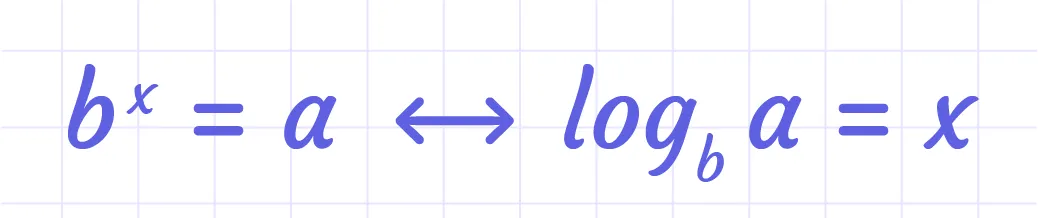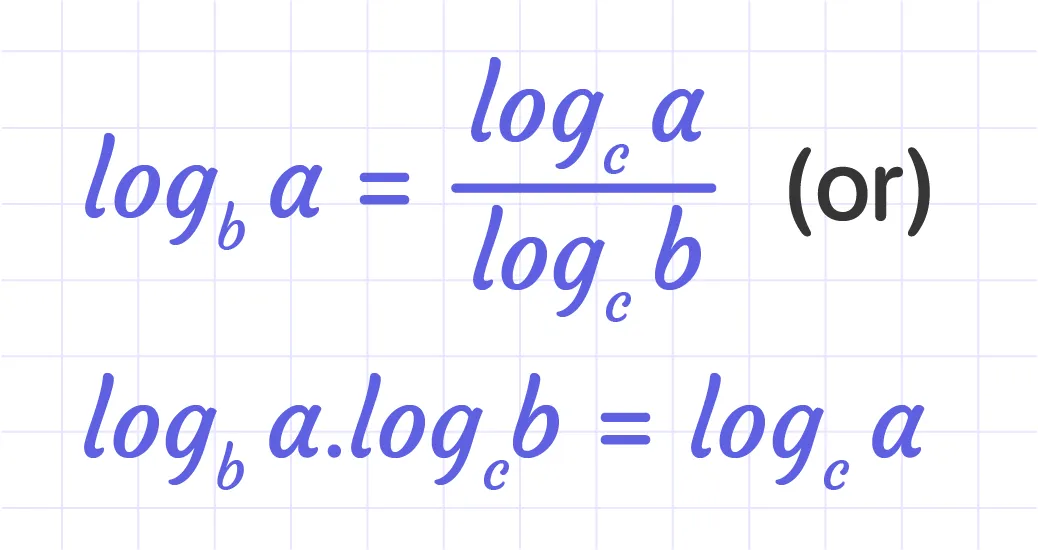# MathMaster Blog

An exponential equation is one with exponents in which the exponent $or a part of the exponent$ is a variable. Exponential equations are classified into three categories:

• with the same bases on both sides: 4^x = 4^2
• with different bases can be made the same: 4^x= 16 which can be written as 4^x = 4^2
• with different bases that cannot be made the same: 4^x = 15.

When the bases on both sides of the equation are not the same, we use logarithms to solve the exponential equations.

For example, neither the bases on both sides of 5^x = 3 are the same, nor can the bases be made the same. In such instances, one of the following options is available.

In these instances, we have such options:

1. Using the given formula we can transform the exponential equation to logarithmic form and then solve it for the variable.2. Use logarithm $log$ on both sides of the equation and solve for the variable. In this case, we have to apply:## Example:

If we turn 5^x = 3 into the logarithmic form, we get log 5^3 = x. Applying the change of base property formula,We have x = $log 3$/$log 5$.